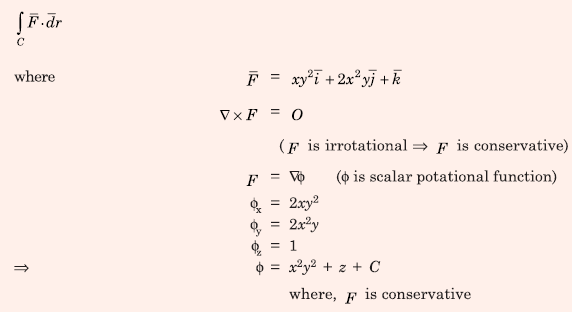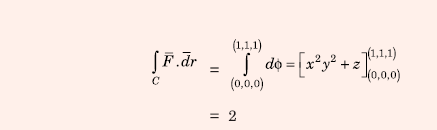# Electromagnetic Fields - Online Test

Q1. Given a vector field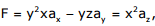the line integral ∫ F.dl evaluated along a segment on the x-axis from x=1 to x=2 is
Explaination / Solution:
No Explaination.

Q2. The curl of the gradient of the scalar field defined by V = 2x2y + 3y2z + 4z2x is
Explaination / Solution:
No Explaination.

Q3. The flux density at a point in space is given by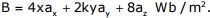The value of constant k must be equal to
Explaination / Solution:
No Explaination.

Q4. A dielectric slab with 500mm x 500mm cross-section is 0.4m long. The slab is subjected to a uniform electric field of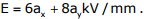. The relative permittivity of the dielectric material is equal to 2. The value of constant ε0 is 8.85 × 10-12 F/m. The energy stored in the dielectric in Joules is
Explaination / Solution:
No Explaination.

Q5. The magnitude of magnetic flux density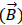at a point having normal distance d meters from an infinitely extended wire carrying current of l A is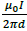(in SI units). An infinitely extended wire is laid along the x-axis and is carrying current of 4 A in the +ve x direction. Another infinitely extended wire is laid along the y-axis and is carrying 2 A current in the +ve y direction µ0 is permeability of free space Assumeto be unit vectors along x, y and z axes respectively.Assuming right handed coordinate system, magnetic field intensity,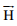at coordinate (2,1,0) will be

Explaination / Solution: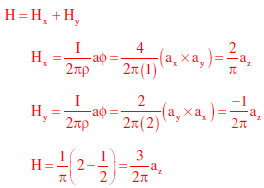Q6. Match the following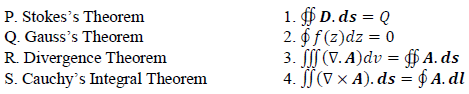Explaination / Solution:
No Explaination.

Q7. Two semi-infinite dielectric regions are separated by a plane boundary at y=0. The dielectric constant of region 1 (y<0) and region 2 (y>0) are 2 and 5, Region 1 has uniform electric field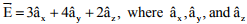are unit vectors along the x, y and z axes, respectively. The electric field region 2 is
Explaination / Solution:Q8. In cylindrical coordinate system, the potential produced by a uniform ring charge is given by φ = f(r, z), where f is a continuous function of r and z. Letbe the resulting electric field. Then the magnitude of ∇ ×Explaination / Solution: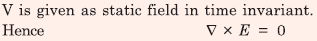Q9. Two electric charges q and −2q are placed at (0,0) and (6,0) on the x-y plane. The equation of the zero equipotential curve in the x-y plane is
Explaination / Solution: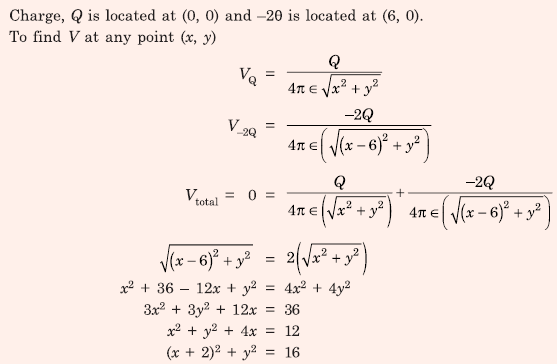Q10. The value of the line integral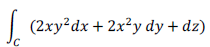along a path joining the origin (0, 0, 0) and the point (1, 1, 1) is
Explaination / Solution: